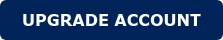Method for Accurately Predicting Core Losses Using Deep Learning

A new method for predicting ferrite properties, particularly core loss density, is proposed.

Through the usage of this method, core loss in power transformers and inductors can be predicted with little use of measuring equipment, only in the training phase. Improvement on these predictions is done with the measurement at speciﬁc points with the help of deep learning methods. The architecture is described and the loss versus frequency, temperature, and peak magnetic ﬁeld graphs from a ferrite material are processed. Finally, an inductor is built and measured, and its loss compared with the one predicted by the proposed method.

1. The main concern about the design of magnetic elements is originated from the non-linearity of the equations and their coupling.

Properties of ferromagnetic materials are difﬁcult to model, although many attempts have been made in the past. The most extended among manufacturers is the Steinmetz loss coefﬁcients  for sinusoidal waveforms, and many researchers have tried to improve these models for concrete cases: Modiﬁed Steinmetz Equation (MSE) , Generalized Steinmetz Equation (GSE) , and improved GSE (iGSE) , but all of these models are based on the Steinmetz coefﬁcients, and these coefﬁcients are a logarithmic regression from real measurements.

Together with these coefﬁcients, manufacturers provide the measurements done in their facilities and produce two-dimensional diagrams that can introduce huge errors when the design point is not close to the sampled parameters.

The recent years have also seen large developments in the ﬁeld of Artiﬁcial Intelligence , and their applications have multiplied. In this paper, a Deep Learning architecture is proposed to solve the problem in obtaining the loss in arbitrary points when designing magnetic components, using only the data provided by the manufacturers and a few lab measurements.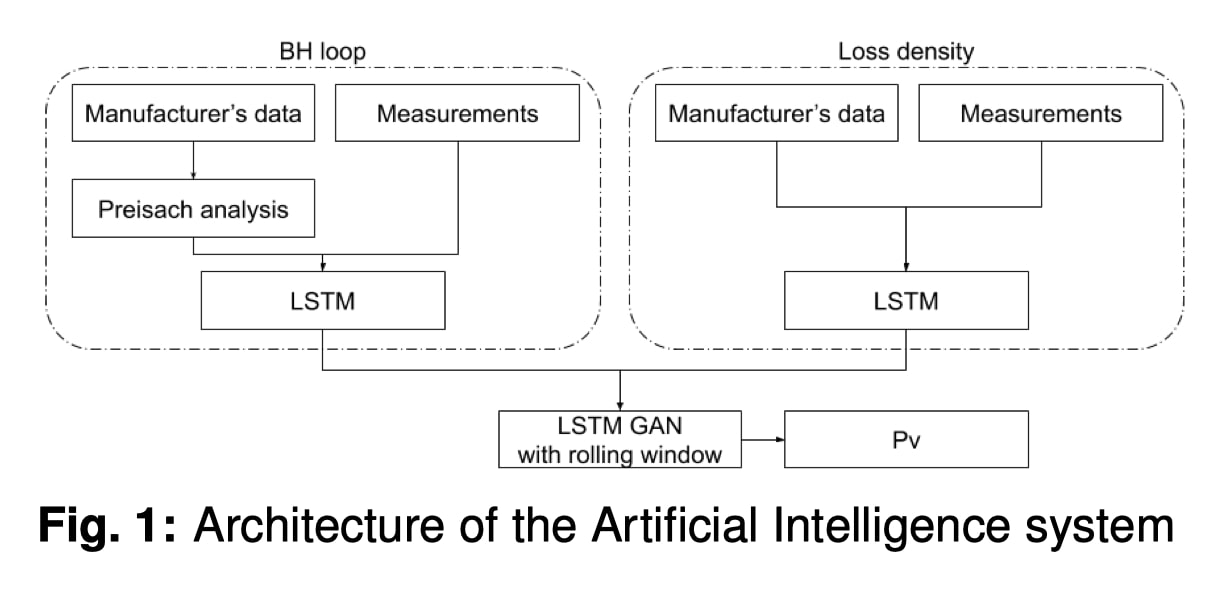2. Architecture of the Artificial Intelligence System

The diagram of the general architecture is shown in Fig. 1. The BH loop section learns from both the measurements and the data provided at different frequencies and temperatures. This information is ﬁrstly processed with various mathematical models, such as normalization, regularization, neighbor-based data cleansing, and sensitivity analysis. Additionally, data provided for loss density includes measurements at different peak magnetic ﬁelds.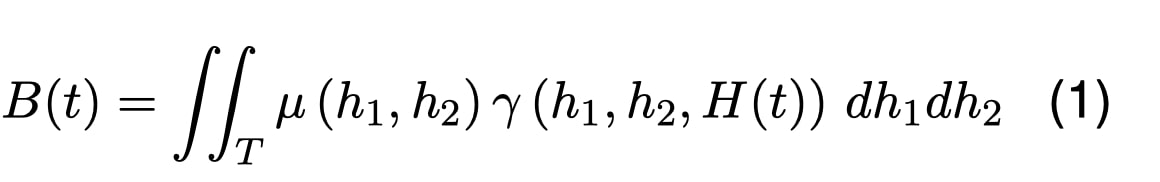Initially, new points from the BH loop are interpolated with cubic splines between the available points to start the Preisach analysis. Once enough points have been calculated, multiple parameters are determined in order to solve the Preisach model (Eq. (1)) in its static form, speciﬁed in Eq. (2) and Eq. (3) from  and :

– m-h coefﬁcients: which take into account the basic behavior of the hysteresis loop.

– Preisach coefﬁcients: calculated from the parameters above, they are a hyperbolic solution of Everett’s formula (Eq. (4)) in its closed form (Eq. (5)). A rate-dependent hysteresis model as in Eq. (6) is used (, , , and ).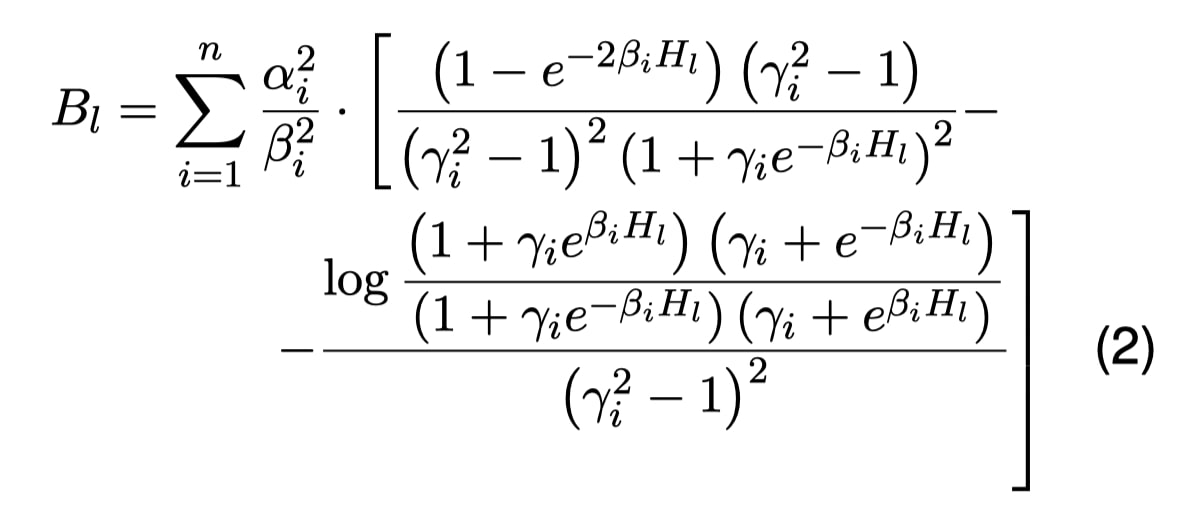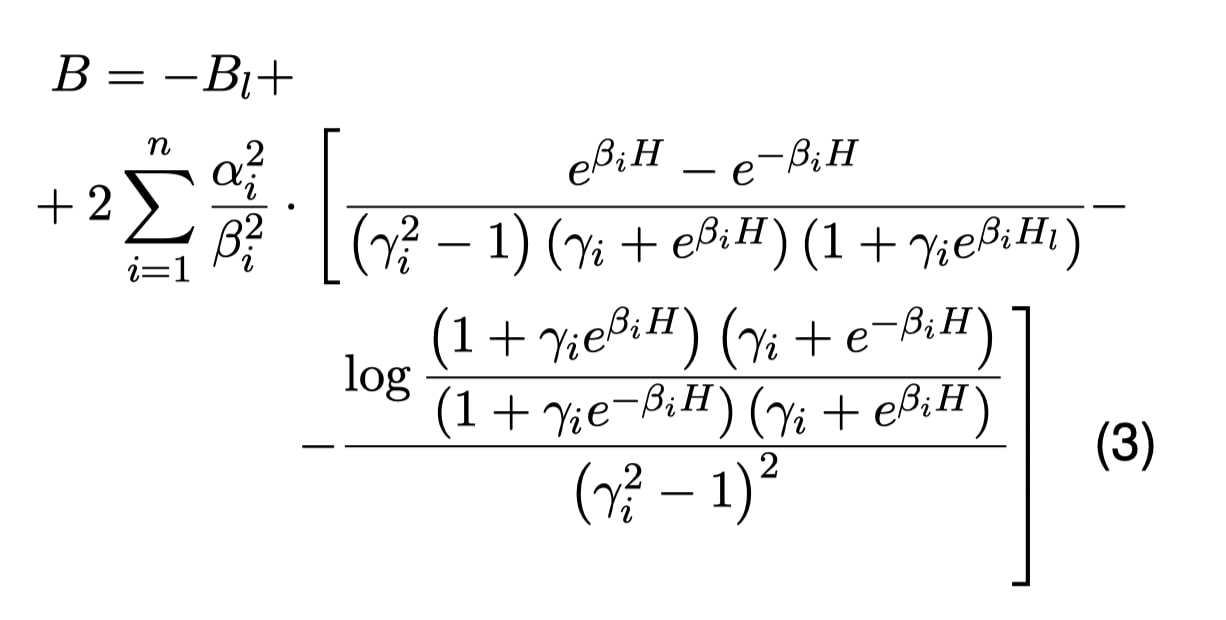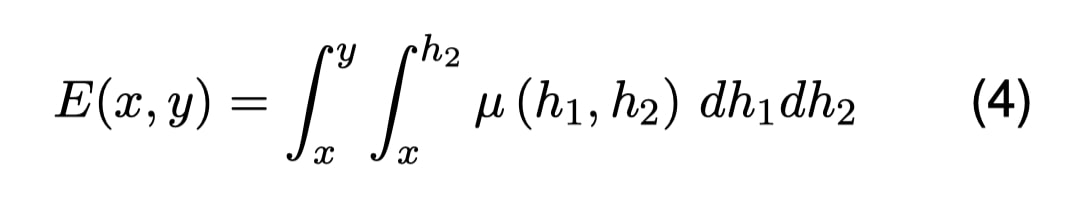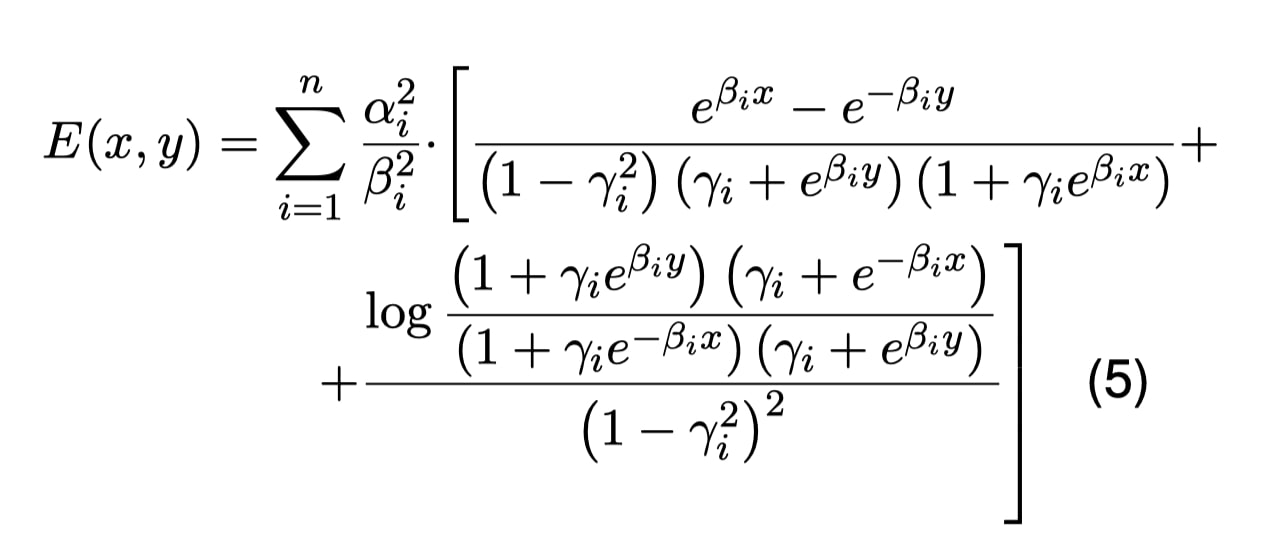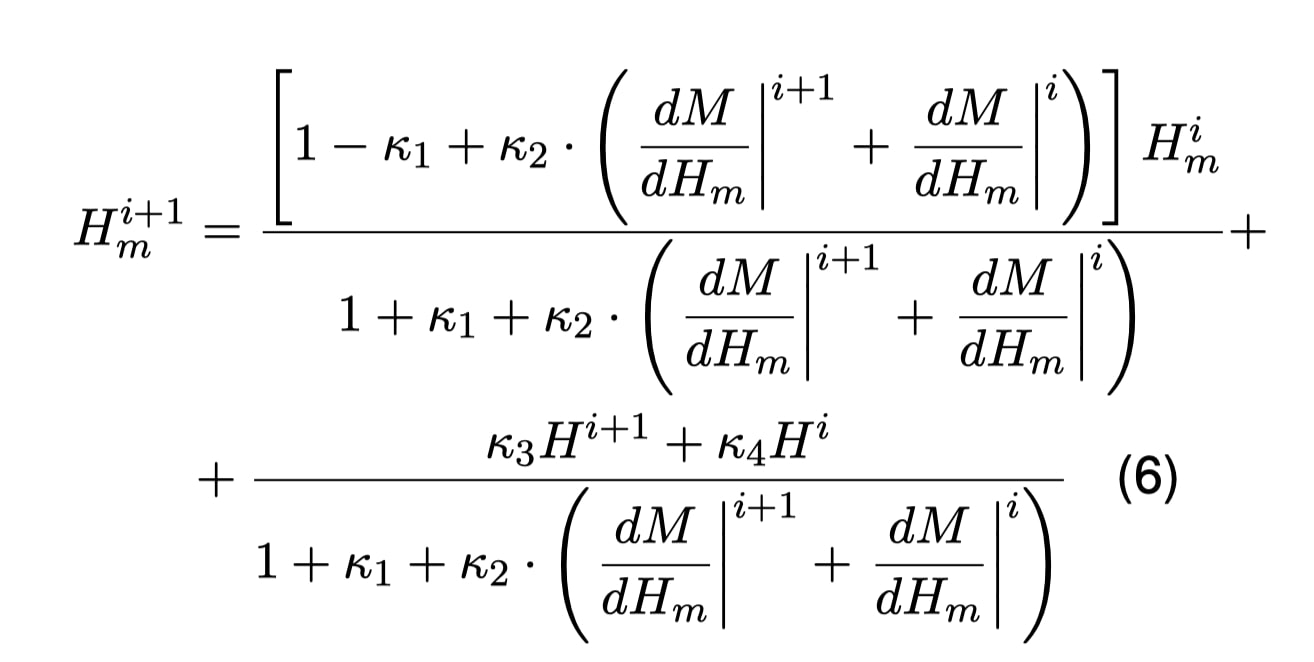From the Preisach coefﬁcients, it is direct to calculate the hysteresis loop at different excitation frequencies. The results at various frequencies and temperatures are unfolded. This way, it becomes easier for the neural networks to process the data: from a BH loop (Fig. 2), a function formed by the positive slope half followed by the negative slope part is produced (Fig. 3).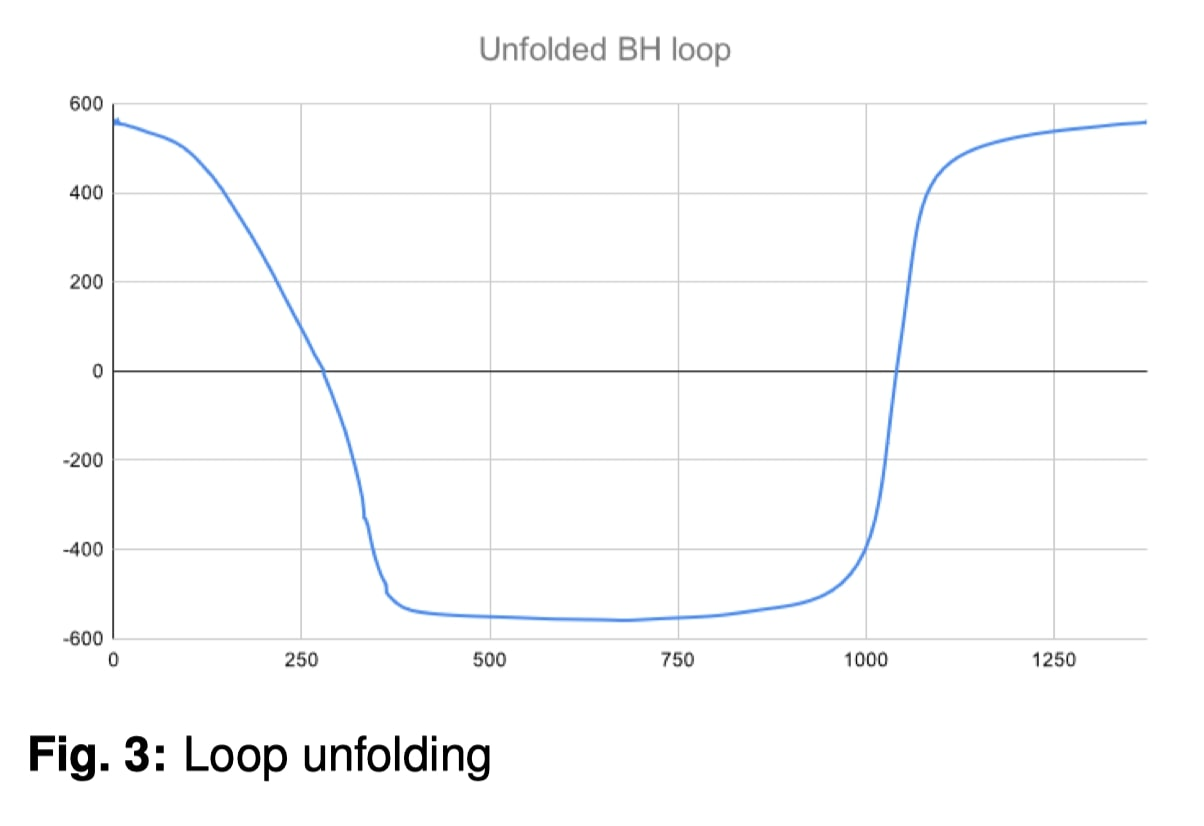These results are then plugged into a recurrent neural network in the form of a long short-term memory (LSTM)  to replicate the behavior at other temperatures. It is trained with a double backpropagation using genetic algorithms , which help the network apply the learned behavior into unexplored regions.

The LSTM block has an internal data preparation structure based on the study of the variables that affect the material behavior with the imposed conditions. Initially, data processing is divided into two channels under the same conditions.On the one hand, the constant temperature channel, where the behavior regarding the growth of ﬂux density (B), magnetic ﬁeld (H), and the magnetization (M), and how different materials behave at different frequencies is studied through the use of neural networks.

On the other hand, there is a constant frequency channel and a range of measurements at different temperatures where the study of the same variables as in the ﬁrst channel is carried out, under these new conditions. The example with saturation is shown in Fig. 4, where it can be seen how the prediction adjusts to the measured data.

With this data, the inputs in the growing neural network (GNN) are conﬁgured and then a set of hysteresis loop solutions at different frequencies and temperatures in the respective working channels are obtained.

After these steps, there are unfoldings with different frequencies at a speciﬁc temperature on one side, and different temperatures at a given frequency on the other. Joining both timelines, a hysteresis mesh (frequency - temperature) is generated, as in Fig. 5, thus forming a prediction taking into account the two dimensions of the hysteresis mesh and all of its conditions.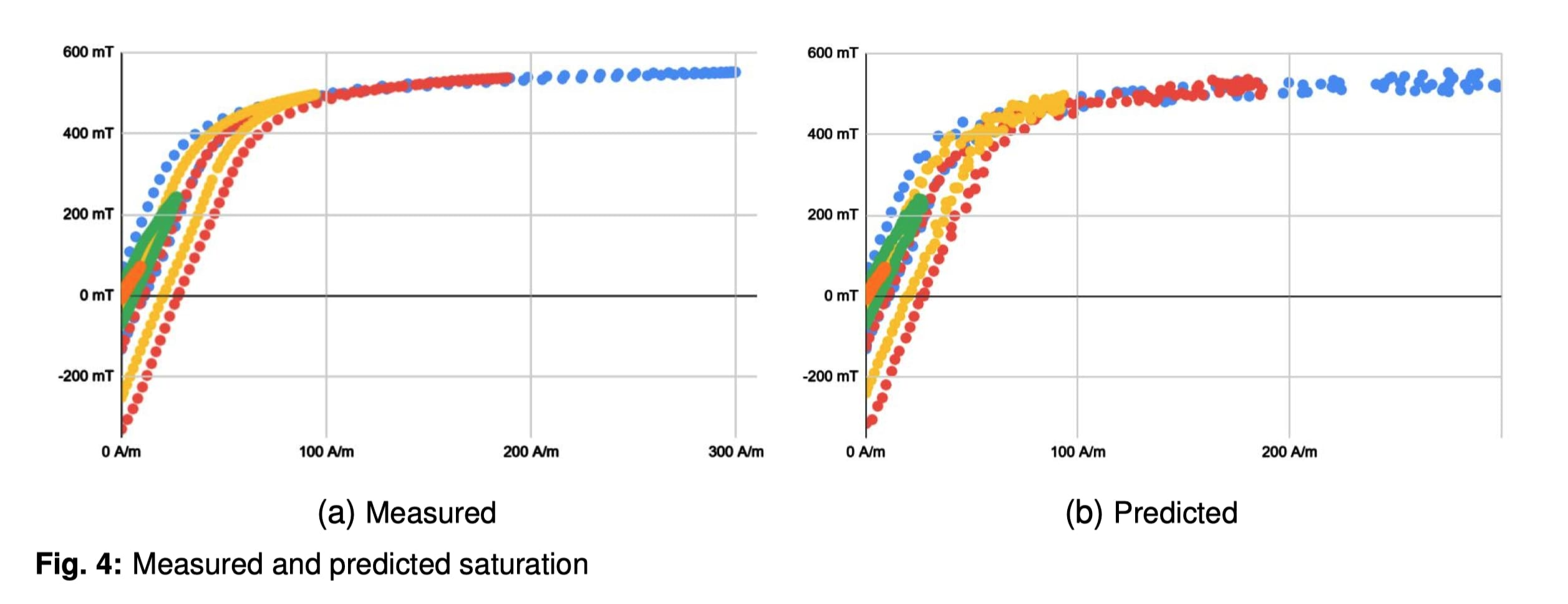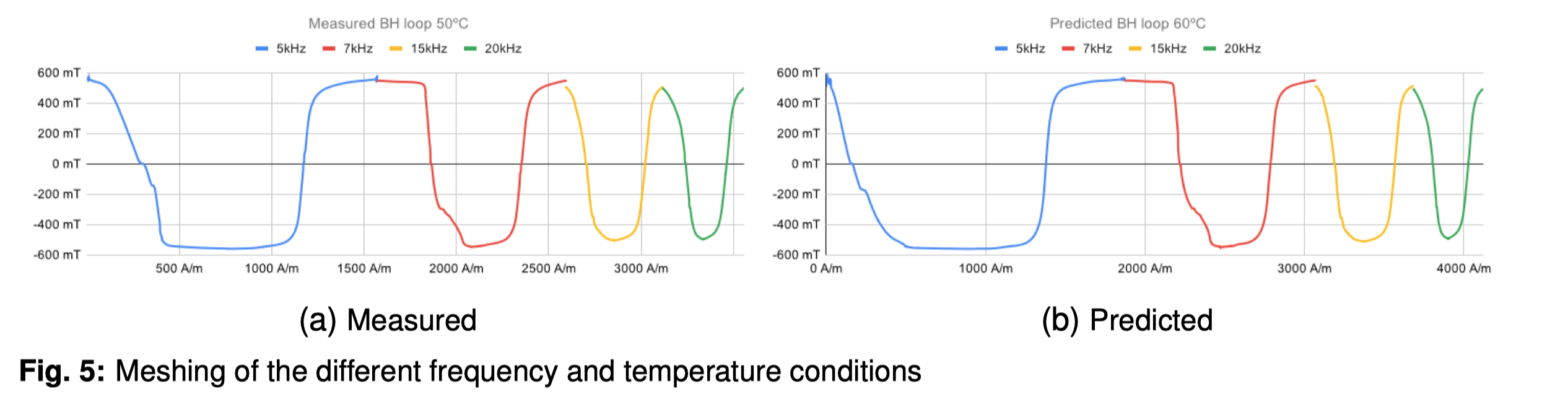From this prediction, an inverse folding is made and the desired hysteresis loop obtained, as shown in Fig. 6.

Finally, a generative adversarial network (GAN)  using LSTM with a rolling window (Fig. 7) is used to discard the points that do not meet the required level of precision as in Fig. 8.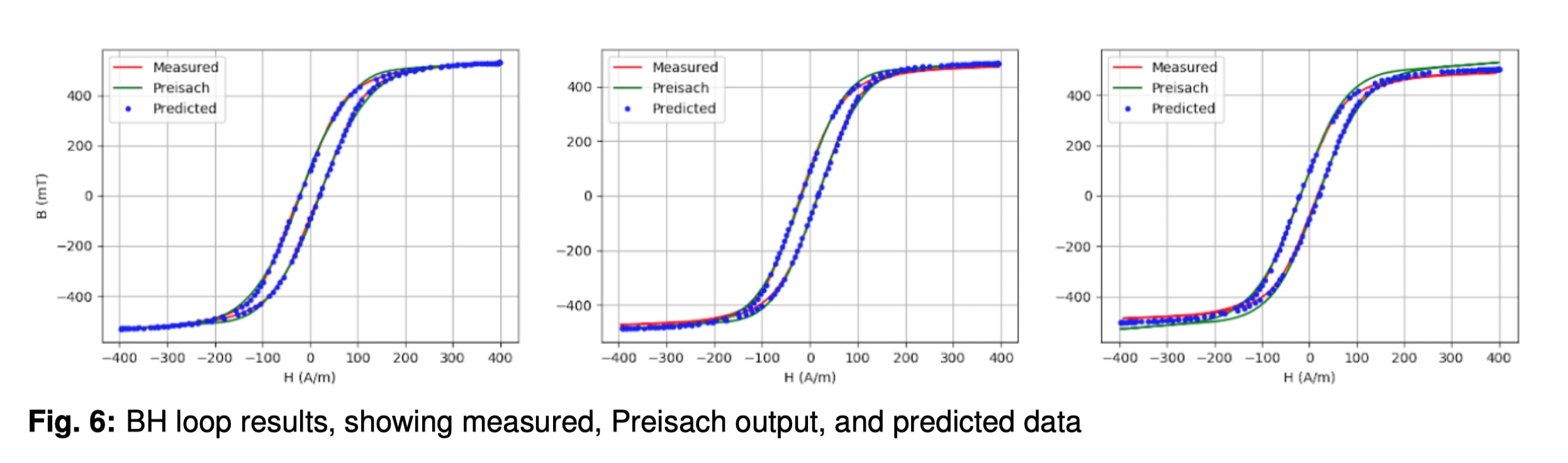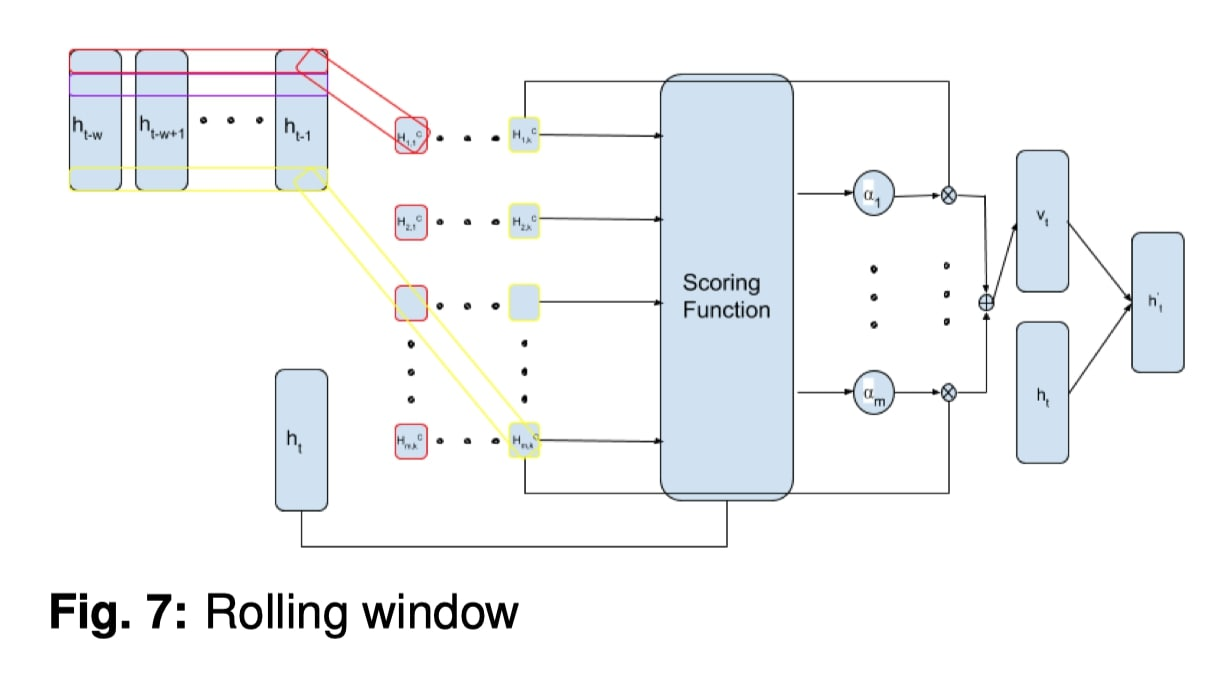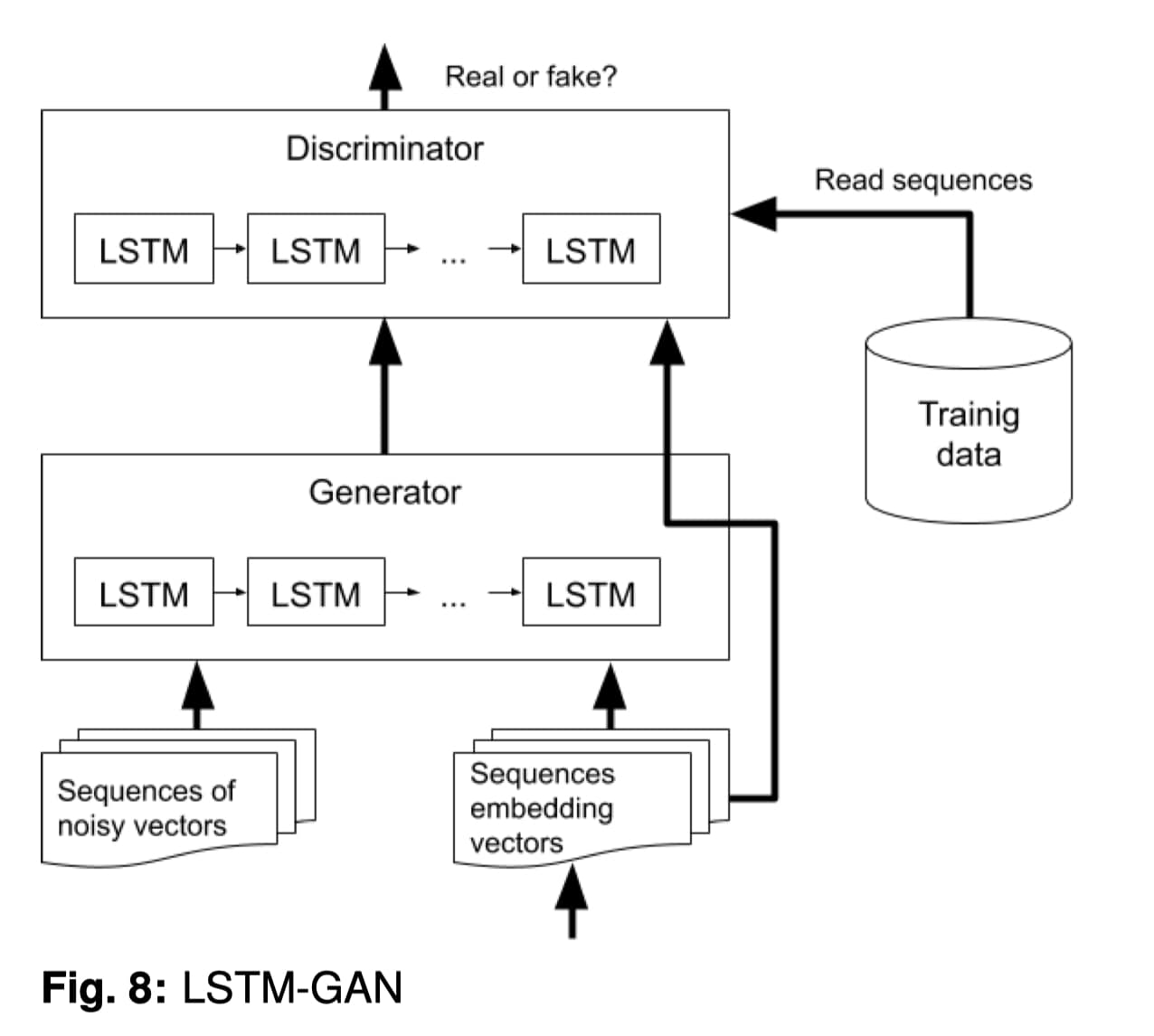The LSTM LGAN (loss GAN) has a slightly simpler structure but is similar to the LSTM HGAN (hysteresis GAN) regarding its effects. The data processing is reduced as it is dependent on the lab measurements or data provided by the manufacturer.

The eddy losses modeling is done following Tak´acs book , but they are negligible in this frequency range.

3. Training is done with both the manufacturers’ data and the measured loops and core loss.

As there are two recurrent neural networks in the architecture, two different pieces of training must be done.

On the one hand, the BH loop LSTM is trained by using the unfolded loop as a time series . As a recurrent neural network, it learns from previous states, so the interpretation as a time series mesh speeds up its training .

On the other hand, the power loss density LSTM is trained from the error between the measured data and the predictions of the ﬁrst LSTM loops. The power is calculated through the magnetic energy integral in Eq. (7).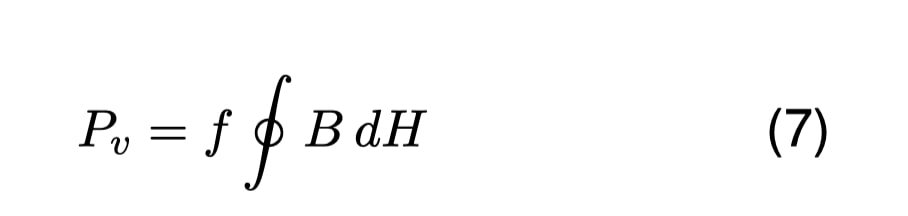For the growth neural network (GNN) shown in Fig. 9, the data provided by ferrite manufacturers is used to calculate the material factor and to extract its behavior from the material’s descriptive variables. From its behavior, our Preisach dynamic model is used to expand the dataset to be veriﬁed with the measurements done in the laboratory. Through this process, the ﬁrst part of the dataset is generated, which is joined by a second part that consists of identifying variables of the material and condition of each cycle. With this data, the GNN is trained. The same process is carried out by the two different work branches in the ﬁrst stage of our LSTM HGAN.

The fusion of the two datasets, maintaining the mesh data architecture as shown in the diagram in Fig. 9, in addition to the conditional variables obtained by each branch of the algorithm, are associated as inputs to each of the meshes. With the prepared data, a hysteresis loop is obtained as a prediction by using the rolling window in our LSTM GAN, with which the parameters of the material and its behavior are taken into account.

The training of the LSTM LGAN (Fig. 10) is more costly in resources because obtaining precise measurements is expensive and difﬁcult. Our dataset is formed by the data provided by manufacturers and, in regions that are considered reliable and under severe constraints, the calculated loss making use of Steinmetz and Jiles-Atherton models are used .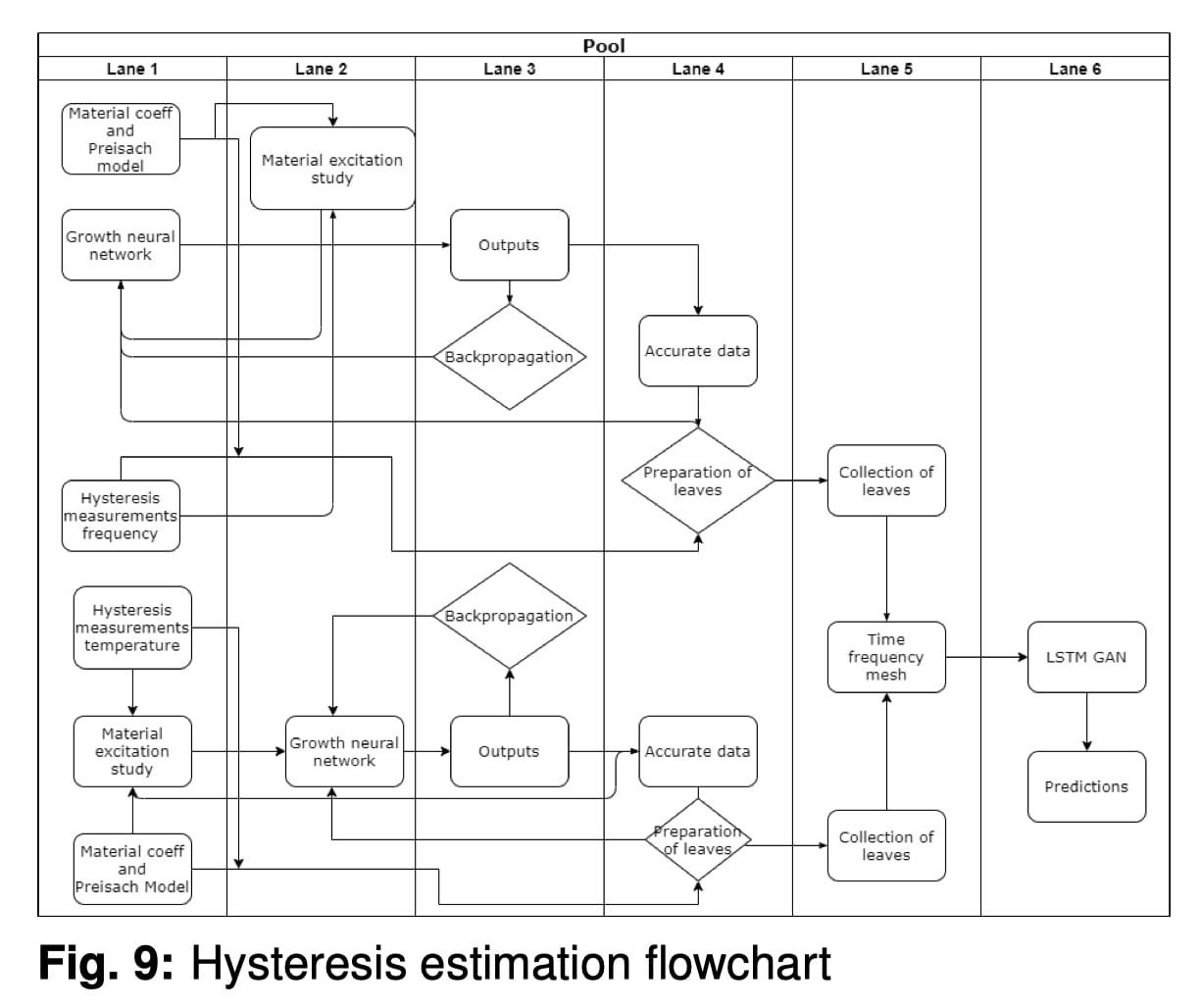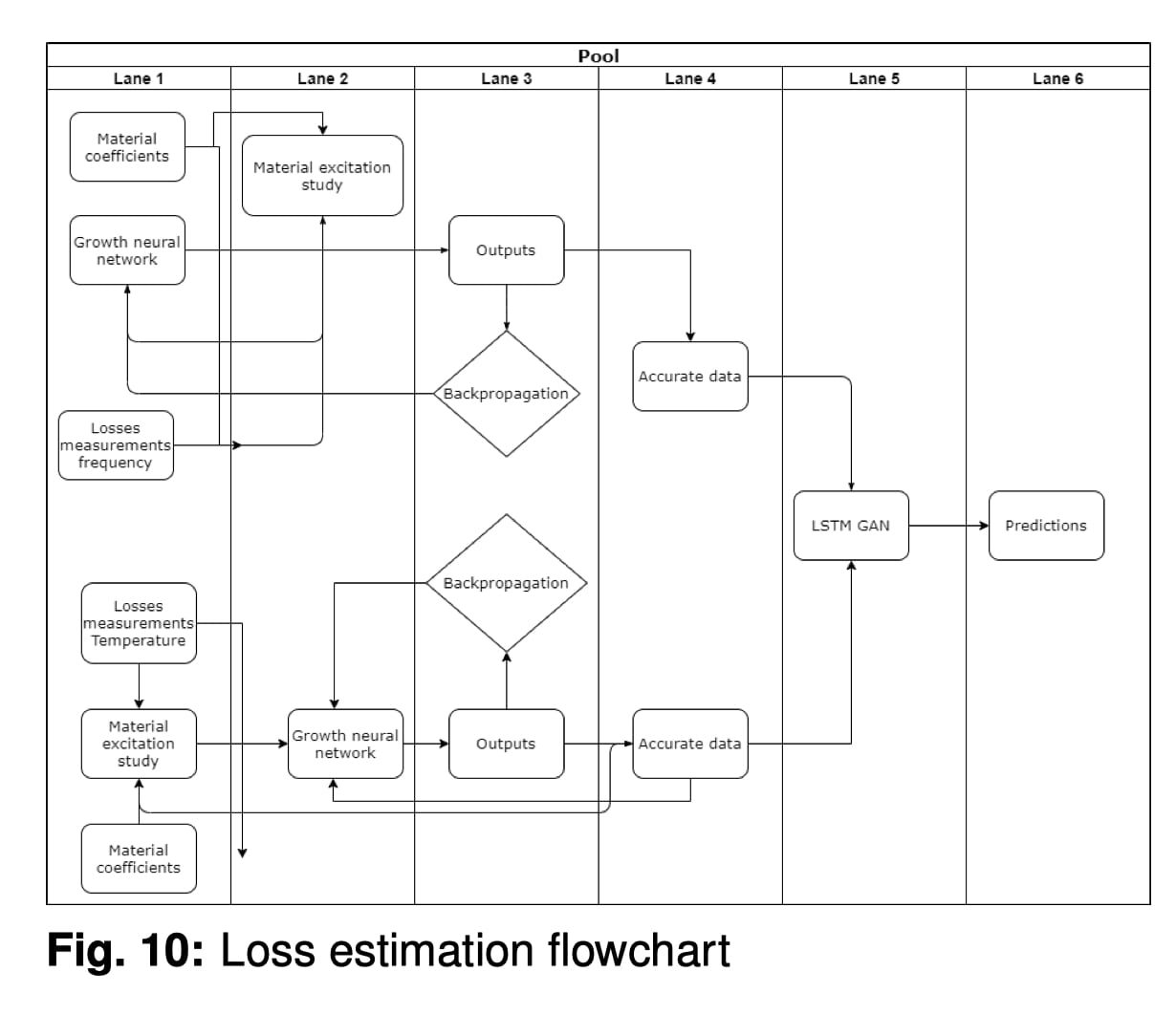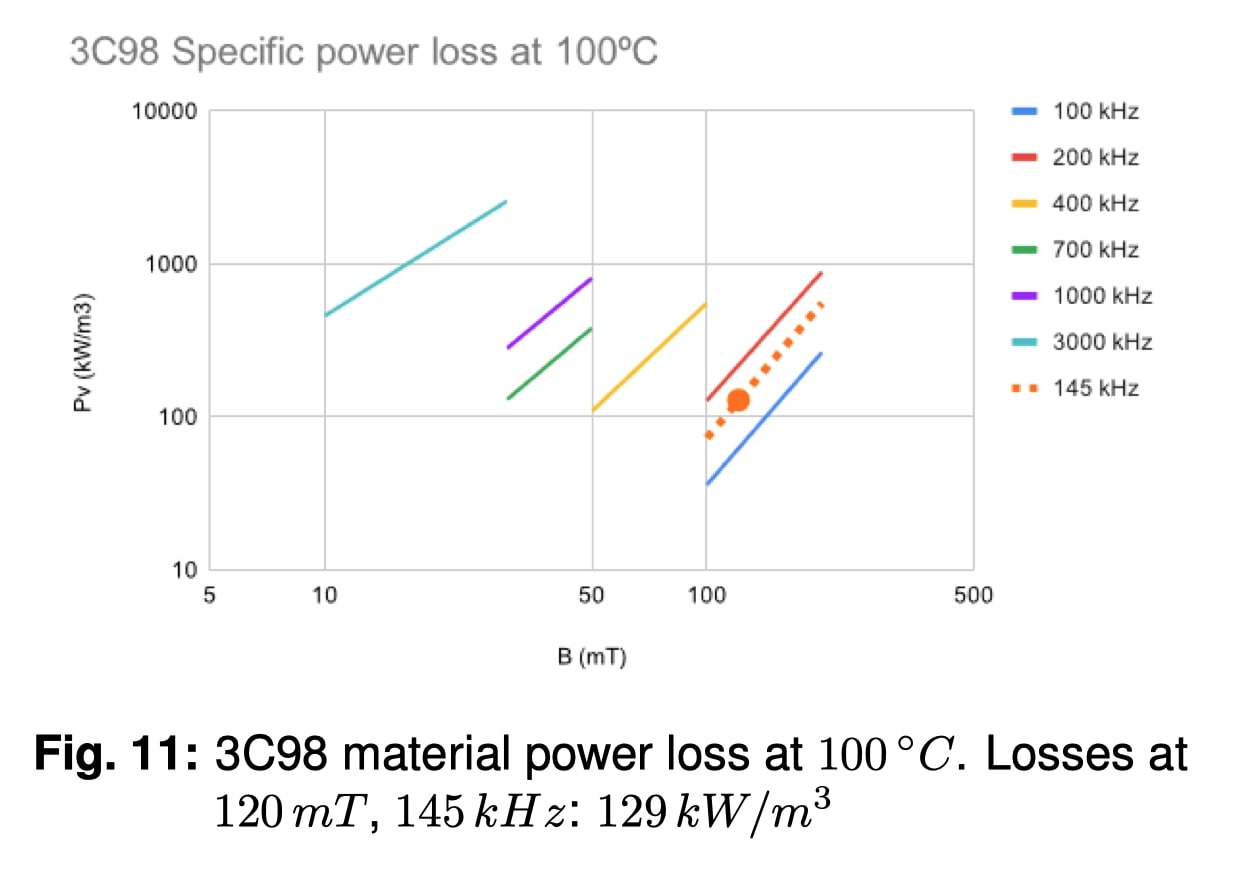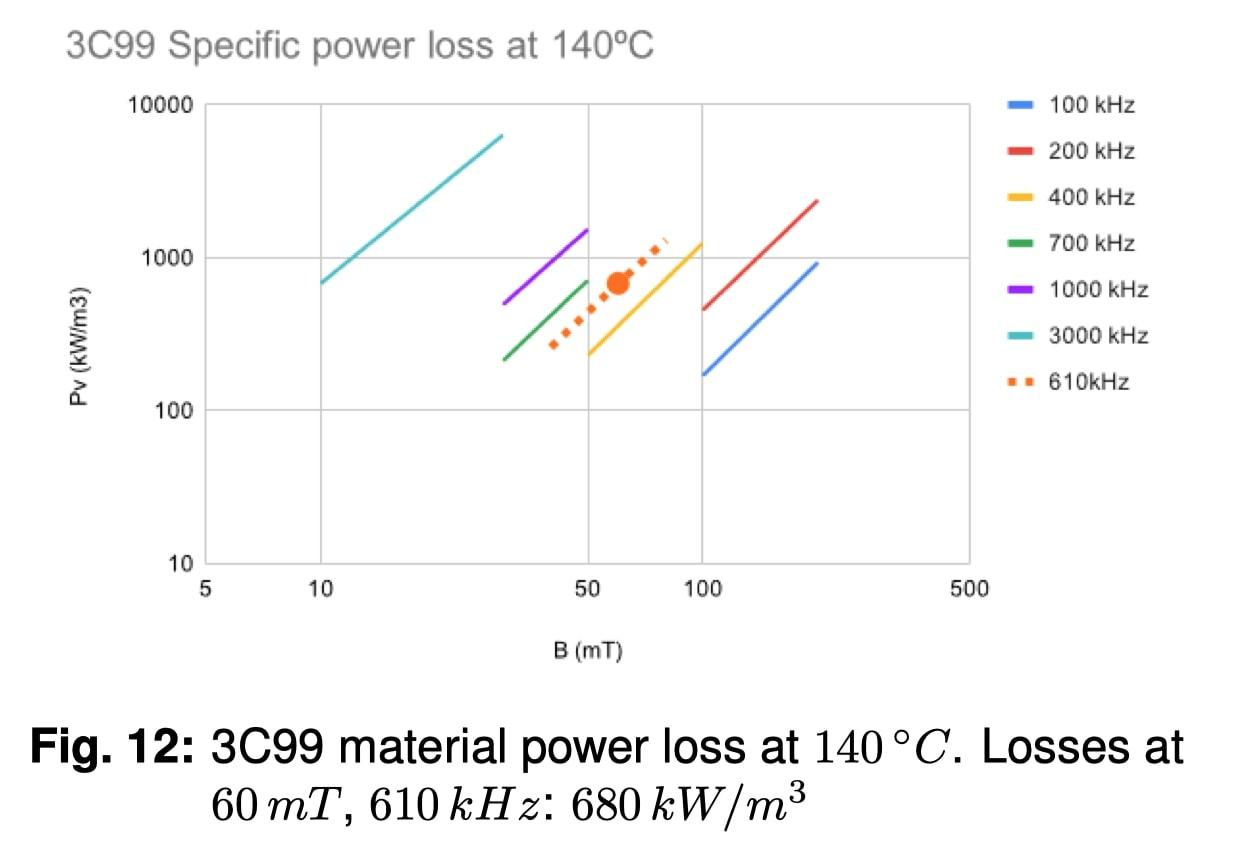4. Experimental validation

Empirical validation was carried out with the testing of an ungapped ferromagnetic toroid made of four materials from Ferroxcube: 3C90, 3C98, 3C99, and 3F36.

For data consistency, the selected toroid was T25/15/10, as the available curves were measured with the same shape. Different turns (5, 10, and 20) were wound.

For the BH loop and power density measurements, a BST-Port-A from Bs & T Frankfurt am Main GmbH was used and a customized circuit with an ad hoc analog integrator for the magnetic ﬂux density measurement.

Power loss results are shown in Fig. 11 and Fig. 12, and BH loops at different temperatures and frequencies in Fig. 13.

In table 1, the error for different excitations is shown.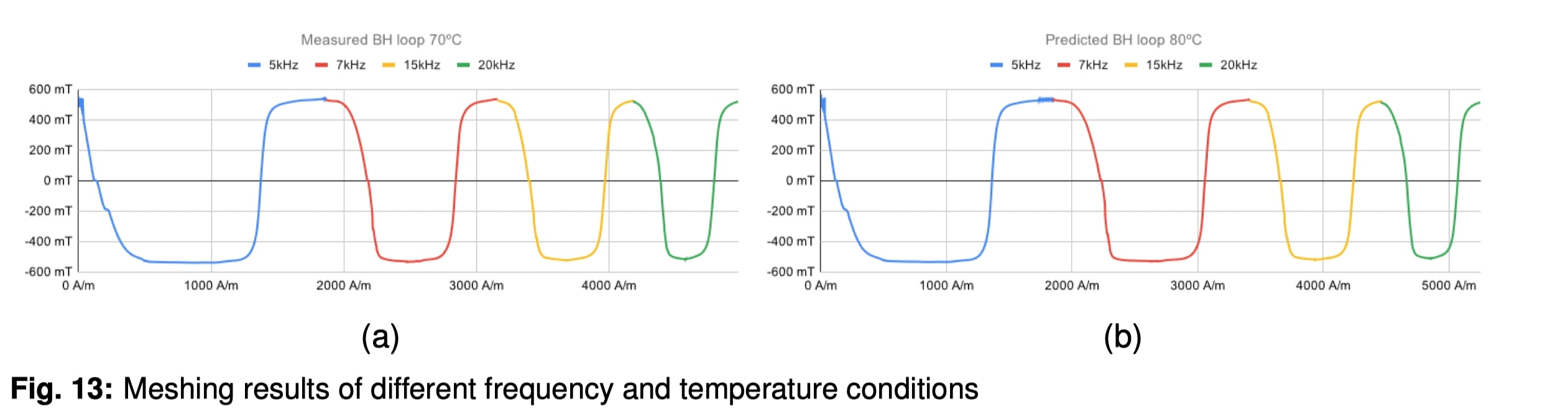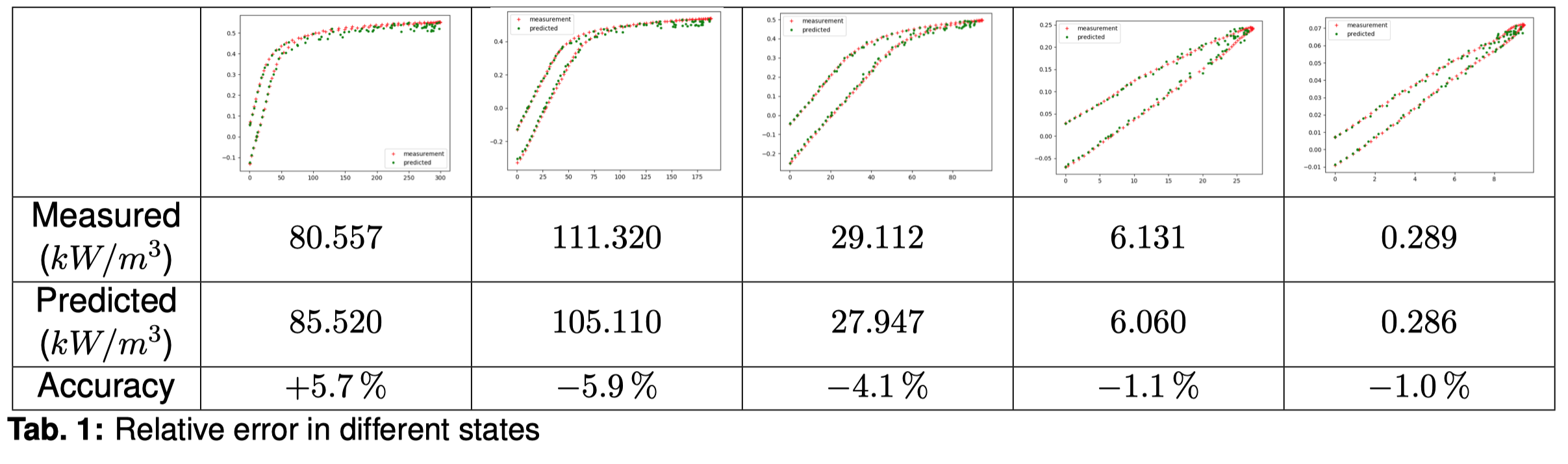It can be observed that there is more error when the material saturates or it is very close due to the noise that the measuring equipment introduces. When the material is more relaxed, predictions are better.

5. Conclusions: A method for predicting the speciﬁc power loss in ferromagnetic cores has been designed, implemented, and validated.

The results given by the Artiﬁcial Intelligence keep the error low due to its intrinsic understanding of non-linearities existing in the materials, in addition to the blending of measurements and analytical models from which it learns.

Predicting power loss with such high accuracy enables engineers to optimize the design of magnetic components by letting them get closer to the working limits of the materials, resulting in more compact and efﬁcient power systems.

The computational nature of this method also enables its integration in design programs, automating the design of power systems without the need for human interaction or experience. In the future, this method will be expanded to other properties of ferromagnetic materials, like permeability and core shapes.

6. Acknowledgement

We would like to thank Ferroxcube for their great assistance in providing the data used for the training of our models.

References

 C. P. Steinmetz, “On the law of hysteresis,” Transactions of the American Institute of Electrical Engineers, vol. IX, no. 1, pp. 1–64, 1892. DOI: 10.1109/T-AIEE.1892.5570437.

 J. Reinert, A. Brockmeyer, and R. De Doncker, “Calculation of losses in ferro- and ferrimagnetic materials based on the modiﬁed steinmetz equation,” Industry Applications, IEEE Transactions on, vol. 37, pp. 1055 –1061, Aug. 2001. DOI: 10.1109/28.936396.

 Jieli Li, T. Abdallah, and C. R. Sullivan, “Improved calculation of core loss with nonsinusoidal waveforms,” in Conference Record of the 2001 IEEE Industry Applications Conference. 36th IAS Annual Meeting (Cat. No.01CH37248), vol. 4, 2001, 2203–2210 vol.4. DOI: 10.1109/IAS.2001.955931.

 K. Venkatachalam, C. R. Sullivan, T. Abdallah, and H. Tacca, “Accurate prediction of ferrite core loss with nonsinusoidal waveforms using only steinmetz parameters,” in 2002 IEEE Workshop on Computers in Power Electronics, 2002. Proceedings., 2002, pp. 36–41. DOI: 10.1109/CIPE.2002.1196712.

 T. Sejnowski, The Deep Learning Revolution, ser. The MIT Press. MIT Press, 2018.

 Z. Szab´o, “Preisach functions leading to closed form permeability,” Physica Bcondensed Matter - PHYSICA B, vol. 372, pp. 61–67, Feb. 2006. DOI: 10.1016/j.physb. 2005.10.020.

 I. Mayergoyz, “Chapter 1 - the classical preisach model of hysteresis,” in Mathematical Models of Hysteresis and Their Applications, ser. Electromagnetism, I. Mayergoyz, Ed., New York: Elsevier Science, 2003, pp. 1 –63. DOI: https://doi.org/10.1016/B978012480873-7/50002-5.

 J. Lemaitre, “Introduction,” in Handbook of Materials Behavior Models, J. LEMAITRE, Ed., Burlington: Academic Press, 2001, pp. xvii –xviii. DOI: https://doi.org/10.1016/ B978-012443341-0/50001-6.

 J. Tak´acs, “Mathematics of hysteretic phenomena: The t(x) model for the description of hysteresis,” p. 173, Sep. 2003. DOI: 10.1002/ 3527606521.

 S. Hochreiter and J. Schmidhuber, “Long short-term memory,” Neural Computation, vol. 9, no. 8, pp. 1735–1780, 1997. DOI: 10. 1162 / neco. 1997 . 9 . 8 . 1735. eprint: https : //doi.org/10.1162/neco.1997.9.8.1735.

 W. Banzhaf, P. Nordin, R. Keller, and F. Francone, Genetic Programming: An Introduction, ser. Morgan Kaufmann Series in Arti. Elsevier Science, 1998.

 I. Goodfellow, J. Pouget-Abadie, M. Mirza, B. Xu, D. Warde-Farley, et al., “Generative adversarial nets,” in Advances in Neural Information Processing Systems 27, Z. Ghahramani,

M. Welling, C. Cortes, N. D. Lawrence, and

K. Q. Weinberger, Eds., Curran Associates, Inc., 2014, pp. 2672–2680.

 S.-Y. Shih, F.-K. Sun, and H. yi Lee, Temporal pattern attention for multivariate time series forecasting, 2018. arXiv: 1809.04206 [cs.LG].

 Y. Yu and S. Canales, Conditional lstm-gan for melody generation from lyrics, 2019. arXiv: 1908.05551 [cs.AI].

 D. C. Jiles and D. L. Atherton, “Theory of ferromagnetic hysteresis,” Journal of Magnetism and Magnetic Materials, vol. 61, no. 12, pp. 48–60, Sep. 1986. DOI: 10.1016/03048853(86)90066-1.

You may also like